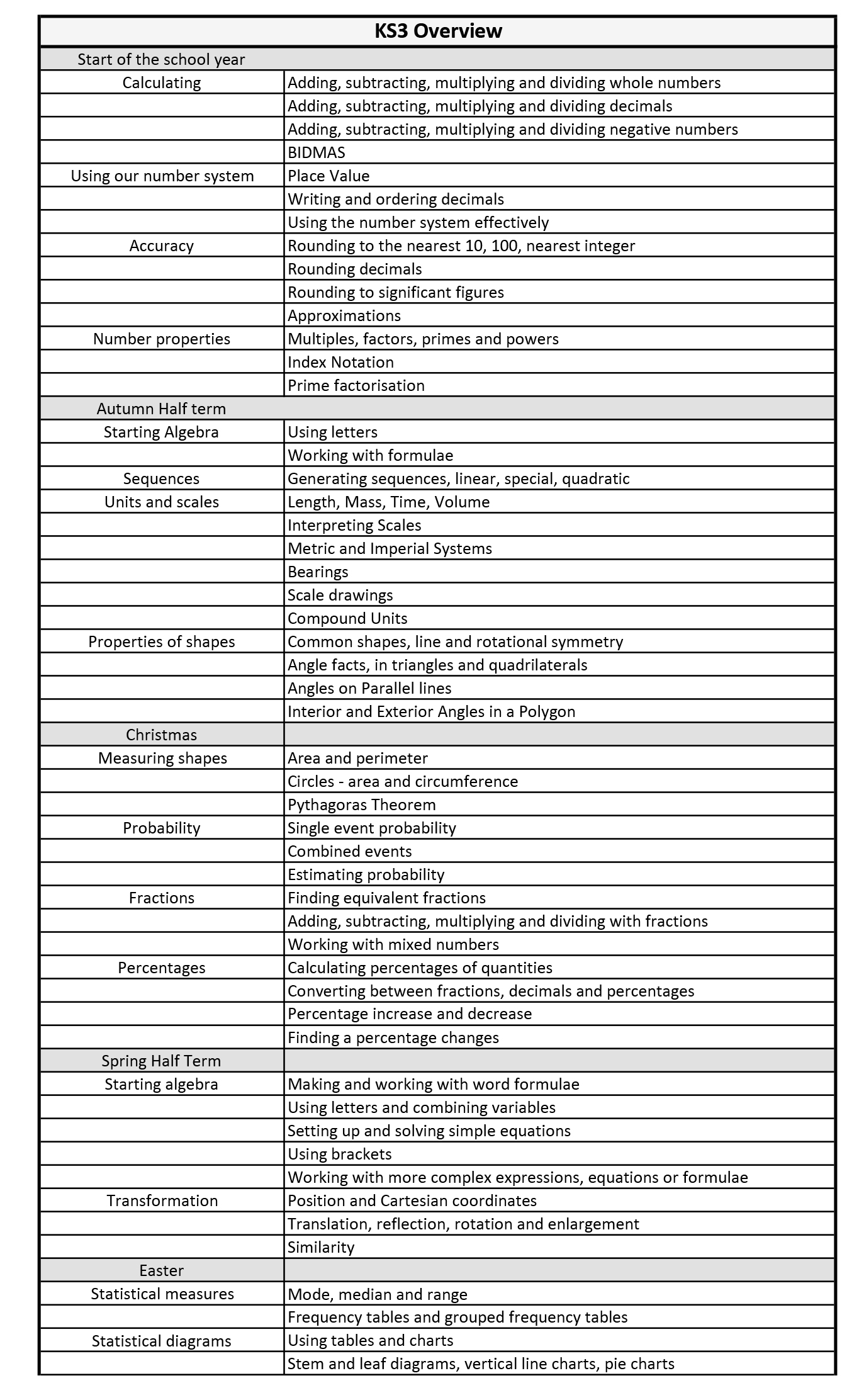## Staff

Ms M Hatfield

Mrs A S Smith
Second in Mathematics

Mrs L Wakefield

Ms C Wood

Miss L Purser

Mrs L Croxton

Miss E O’Sullivan

## Programme of Study – Key Stage 3

Students complete a two year KS3 programme of study in Years 7 & 8.
As soon as the students arrive in Year 7 we conduct a baseline assessment with them. We use this, along with their primary school Mathematics data to inform us of their current ability and they are then grouped accordingly. This allows the teacher to personalise their scheme of work, according to the group.
Assessments are undertaken frequently and amendments to ability groups can take place, throughout the two years.## Programme of Study – Key Stage 4

### Year 10 Overview – Foundation

Number (4 rules for fractions, standard form, percentage, ratio, rounding, direct proportion, identify groups of numbers, HCF, LCM)
Algebra (indices, equations, factorising, sequences to term-to-term rule. Distinguish between equation, formula identity expression, generate and use formulae)
Shapes and Space (co-ordinates, straight-line graphs, construction of triangles, Pythagoras’ Theorem, scales, bearings, area, surface area and volume including circles, transformations, similar and congruent figures, conversion graphs, time-distance graphs, draw angles and lines accurately, convert units including imperial, plans and evaluations, tessellations)
Data Handling (pie charts, bar charts, stem and leaf diagrams, scatter diagrams, time series graphs, probability and use of AND rule, mean, median, mode, range including from tables, sampling, data collection, types of data)

### Year 10 Overview – Higher

(foundation content plus the following)

Number (fractional and negative indices, surds, rational / irrational numbers, standard form problems, inverse percentages, compound interest, density, bounds, finding nth term in sequences, reciprocal)
Algebra (equations including those involving algebraic fractions, factorising quadratics, re-arranging formulae, simultaneous)
Shape and Space (angles in polygons, bearings, properties of polygons, tangents to circles, finding equations of graphs and their perpendiculars, mid-point between 2 co-ordinates solve angle problems, speed and density problems, graphs of quadratics, cubics, reciprocals, exponential and trig functions, trigonometry, angle proofs, constructions, area, surface area, volume including pyramids, cones, frustums and sectors)
Data Handling (lines of best fit, cumulative frequency graphs, histograms, probability of combined events including OR rule, box plots, quartiles, interquartile range, compare distributions, compare merits of different types of sampling methods including stratified sampling, find different averages with grouped data. Know the merits of different averages, cumulative frequency)

### Year 11 Overview – Foundation

Algebra (trial and improvement, finding nth term)
Shape and Space (properties of shapes, angles in polygons)

Examining Board: Pearson Edexcel
Course Outcome: GCSE
http://qualifications.pearson.com/en/qualifications/edexcel-gcses/mathematics-2015.html